# Histograms

Go back to  'Data, Statistics'

## Introduction to Histograms

So far, we have discussed graphs that represent discrete data intervals/set. What do we do when the intervals are continuous? This is where histograms come into the picture as the charts are used to properly illustrate the values for a wide distribution of a variable.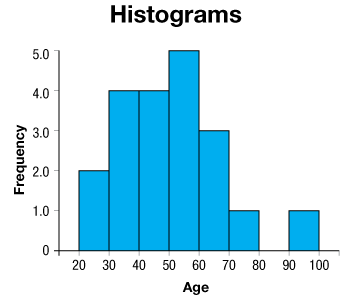Much like the other graphs used in statistical analysis, histograms are a great aid in dealing with closely separated categories of the same variable and can even be drawn upon other histograms to correlate the values with another variable.

## The Big Idea: What are histograms? What are continuous intervals?

Histograms, as the name suggests, are graphs that represent the distribution of the variable in question.

In a histogram, the area of the bar indicates the frequency of occurrences whereas the height of the bar may not necessarily indicate how many occurrences are in each bin.

It is the product of the height and the width of the bin that gives the frequency of occurrences within that bin.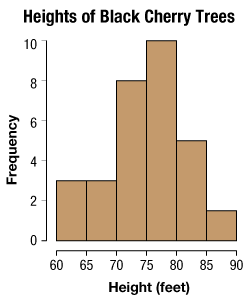One way to understand histograms is by understanding the frequency of the variables that they use. Histograms represent and show a continuous set of values on the horizontal axis and show all possible values of a continuous random variable.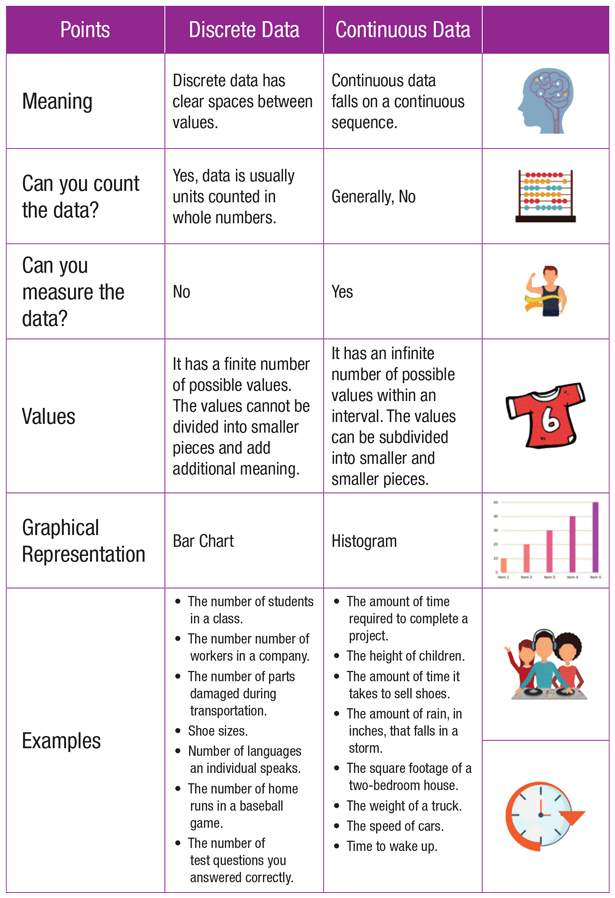A simpler way to understand frequency distributions would be by taking the case of flipping five coins for five experiments and noting the number of heads and tails that are produced with each experiment.

Then by plotting the number of heads and tails on the $${x}$$-axis, the probabilities for the same are plotted on the $${y}$$-axis. The probability values if summed up will produce a frequency distribution for all the values which will successively add to 1.

This is an example of a continuous random variable where the set of possible values (known as the range) is infinite and uncountable.

A frequency distribution is thus a representation, either in a graphical or tabular format, that displays the observations within a given interval.
These intervals are mutually exclusive and exhaustive, and the interval size depends on the data being used.

To convert discontinuous intervals into continuous intervals, understanding the difference between histograms and bar charts is essential.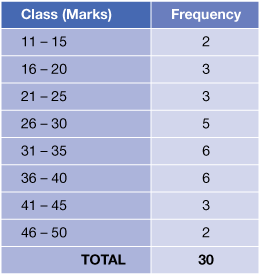Discrete data is the type of data that has clear spaces between values while continuous data has a continuous sequence. Discrete data is graphically represented by bar graphs whereas histograms illustrate continuous data graphically. To convert one into the other, one can:

• Sort the categories from those that occur most frequently to the least occurring.
• Split the intervals between a range (say [0, 1]) into sections based on the cumulative probability for all the category.
• Or find an interval [, ] that relates to the [0, 1] for the categories and represent them on a chart

## How is it important?

### The relationship between a histogram and bar graph

The fundamental difference between histograms and bar graphs from a visual aspect is that bars in a bar graph are not adjacent to each other.

Data in bar graphs are grouped using parallel rectangular bars of equal width but with varying length. Each rectangular block indicates a specific category whose length is influenced by the value of the category. The bars in a bar graph are supposed to represent values for a single variable over various categories and subclasses.

This is not true for the histogram as the area under the curve represents distributive data for a single variable. While other variables can be added as in the case of a multivariate graph, it can become hard to navigate through.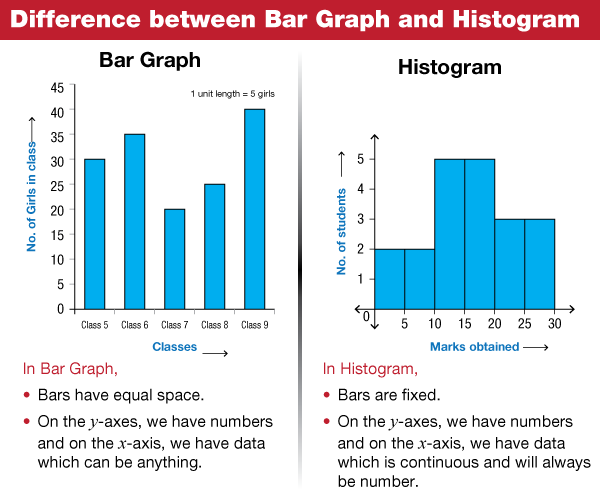As such, a histogram presents numerical data whereas bar graphs show categorical data. Just take a look at the distribution graphs for chi-squares and the simpler z distribution and see that the area under the graph will eventually add up to 1. So, if dealing with datasets that are continuous, use histograms.